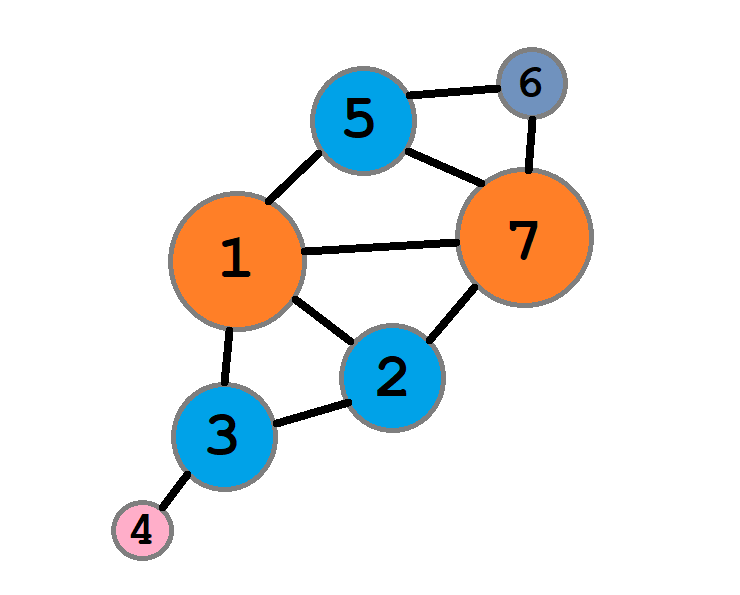시간 제한메모리 제한제출정답맞힌 사람정답 비율
2 초 1024 MB3000.000%

## 문제This molecule corresponds to the graph in the sample.

Unununium (Uuu) was the name of the chemical element with atom number 111, until it changed to Röntgenium (Rg) in 2004. These heavy elements are very unstable and have only been synthesized in a few laboratories.

You have just been hired by one of these labs to optimize the algorithms used in simulations. For example, when simulating complicated chemical reactions, it is important to keep track of how many particles there are, and this is done by counting connected components in a graph.

Currently, the lab has some Python code (see attachments) that takes an undirected graph and outputs the number of connected components. As you can see, this code is based on everyone's favourite data structure union-find.

After looking at the code for a while, you notice that it actually has a bug in it!  The code still gives correct answers, but the bug could cause it to run inefficiently. Your task is to construct a graph with a given number of vertices and edges where the code runs very slowly. We will count how many times the third line (the one inside the while loop) is visited, and your program will get a score according to this number.

## 입력

The input consists of one line with two integers $N$ and $M$, the number of vertices and edges your graph should have. Apart from the sample, there will be only one test case, with $N = 100$ and $M = 500$.

## 출력

The output consists of $M$ lines where the $i$:th contains two integers $u_i$ and $v_i$ ($1 \leq u_i, v_i \leq N$). This indicates that the vertices $u_i$ and $v_i$ are connected with an edge in your graph.

Your graph must not contain any duplicate edges or self-loops. That is, $u_i$ must be different from $v_i$ and all the sets $\{u_i, v_i\}$ must be distinct.

## 점수

Let $X$ be the number of times the innermost while loop is visited when given your test case. Then your score will be $\min(X / 200, 100)$.

You will not get any points for the sample test case.

## 예제 입력 1

7 10


## 예제 출력 1

1 2
2 3
1 3
3 4
5 6
6 7
5 7
1 7
7 2
5 1


## 힌트

In the sample case, the output contains a graph that causes the innermost loop to be visited $20$ times. Run the code and see for yourself!

## 출처• 문제를 만든 사람: Nils Gustafsson

## 채점 및 기타 정보

• 100점 이상을 획득해야 를 받는다.
• 예제는 채점하지 않는다.